Math & Beyond
15 Famous Mathematicians and Their Contributions
567.4k views

 1 Introduction 2 Famous Mathematicians list and their contributions 3 Conclusion

## Introduction

Being a mathematician is hard. Only a few people have mastered this subject and achieved fame. Of those, there have been some famous Indian mathematicians. In this article, we will discuss some of the famous mathematicians and their contributions to Mathematics.

Here is a PDF that consists of riddles about famous mathematicians. Click on the download button to solve them.

## 15 Famous Mathematicians and Their Contributions

### 1. Euclid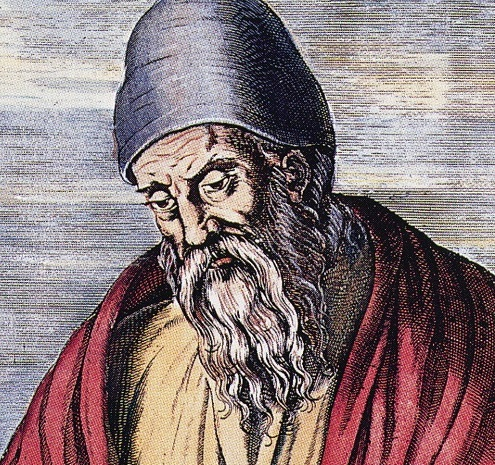Euclid was one among the famous mathematicians, and he was known as the ‘Father of Geometry.’ His famous Geometry contribution is referred to as the Euclidean geometry, which is there in the Geometry chapter of class IX. He spent all his life working for mathematics and set a revolutionary contribution to Geometry.

### 2. Pythagoras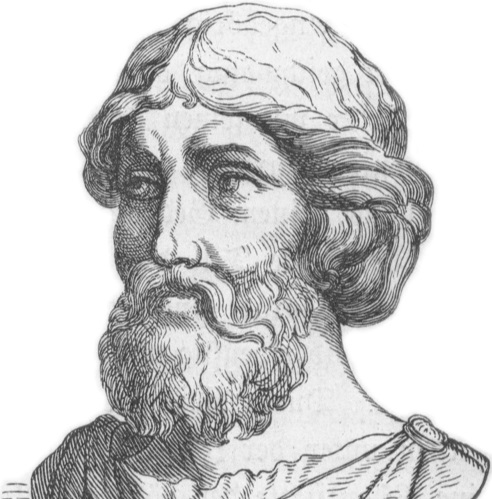‘Pythagoras theorem’ is very popular and an important mathematical formula to solve mathematical problems. Since childhood, all of us have struggled to solve mathematical problems applying the Pythagoras theorem. Pythagoras discovered this prominent theorem and he became the father of ‘Pythagoras theorem.’ Pythagoras was from Greece, but he fled off to India in the latter part of his life.

### 3. Archimedes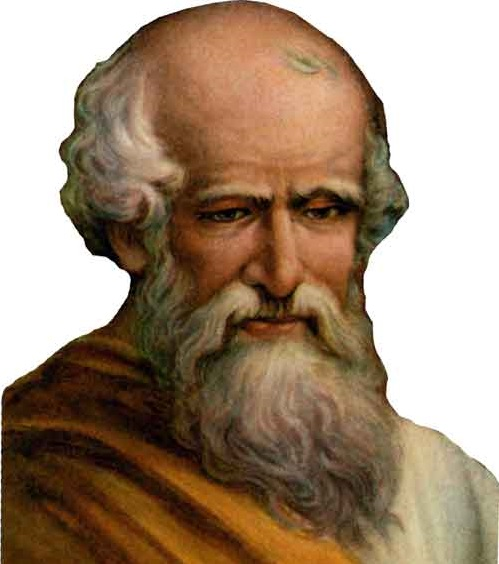Archimedes was a Greek Scientist, a great mathematician, and a Physician. He also worked his entire life in search of discovering mathematical formulas that are related to Physics. The best contribution of Archimedes in mathematics is known as the invention of compound pulleys, antiquity, and screw pump. Students of class X have to study these chapters of compound pulleys and antiquity.

### 4. Thales of Miletus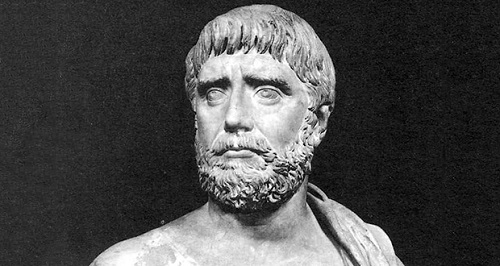Thales of Miletus was one of the most famous mathematicians from Greece. He was very skilled in Geometry and used Geometry as a means to calculate the heights of pyramids and measure the distance of a ship from the shore. As he was also a philosopher, in the ‘Thales’ theorem’ he tries to apply Geometry by using deductive reasoning and derives the conclusion of four corollaries. You can learn more about Thales of Miletus here.

### 5. Aristotle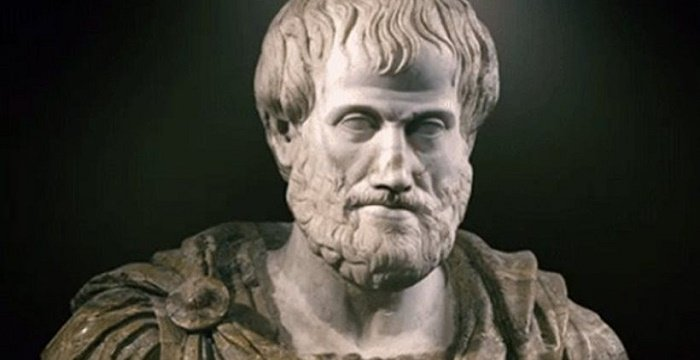Aristotle was a great scholar and he had vast knowledge in various areas, including Physics, mathematics, geology, metaphysics, medicine, biology, and psychology. He was a student of Plato, and both of them together discovered many philosophical theories and contributed to mathematics and Platonism. He combines mathematics and philosophy and in his treaties, and uses mathematical science in three principal ways.

### 6. Diophantus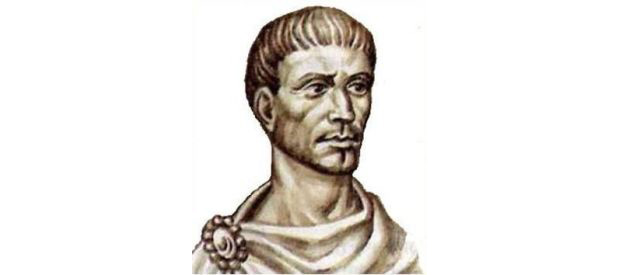His algebraic equations are quite easy and unique, so he was popularly known as the ‘Father of Algebra.’ He wrote a series of books on Algebra. He later gained popularity for his book Arithmetica, where a brief description with examples was given on the best solution for all the algebraic equations and the theory related to the number.

### 7. Eratosthenes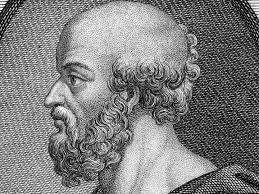Eratosthenes was a world-famous mathematician known for his unbelievable and exact calculation. He was the only mathematician who put efforts to calculate the earth’s circumference and calculated the Earth’s axis tilt. Both his calculations are exact, and so he became famous worldwide.

### 8. Hipparchus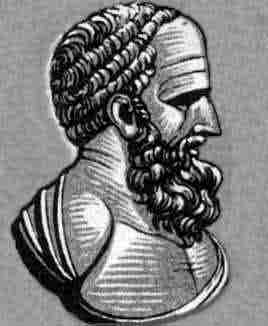Like Geometry, trigonometry chapters are also important for class IX and X students. The founder of trigonometry was an intelligent mathematician and mythologist Hipparchus. He discovered the first trigonometric table in mathematics. He was the first person to develop a well-grounded process by which people can predict solar eclipses.

### 9. Hero of Alexandria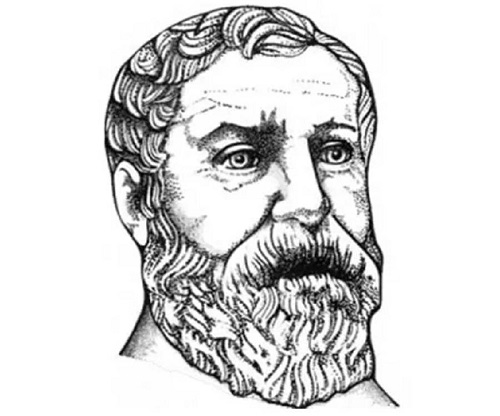Heron’s formula in mathematics is applied by students from class VI onwards. Yes, he was the one who discovered the square root of numbers. In today’s mathematics, his formula is known as Heron’s formula. So, he gained popularity and became known as the ‘Hero of Alexandria.’

### 10. PtolemyPtolemy was a mathematician; he was also a geographer, musician, writer, and astronomer. His contributions to mathematics were incredible. He wrote about mathematics, and among them, his best treaty was called Almagest. He also believed that in the Universe, the position of the Earth was in the center.

### 11. Xenocrates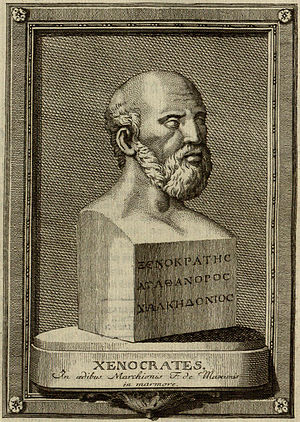Xenocrates was a famous mathematician from Greek. He had written a series of books on mathematics. He emphasizes the theory of numbers in mathematics, and all his written books were based on the theory of numbers, and geometry. He could easily calculate the syllables from an alphabet.

### 12. Anaxagoras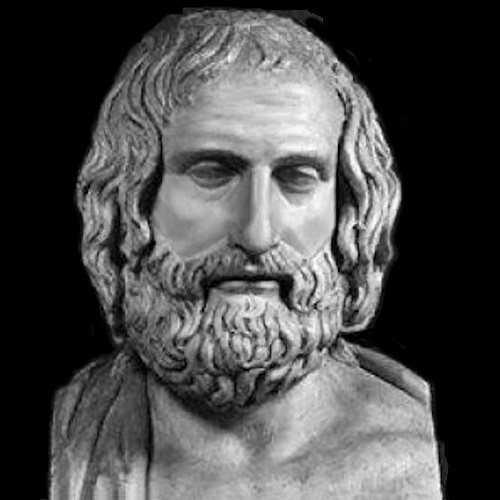He was a great mathematician and an astronomer. Because of his outstanding knowledge of mathematics and cosmology, he discovered the exact clarification of eclipses and stated that the Sun is larger than Peloponnese.

### 13. Hypatia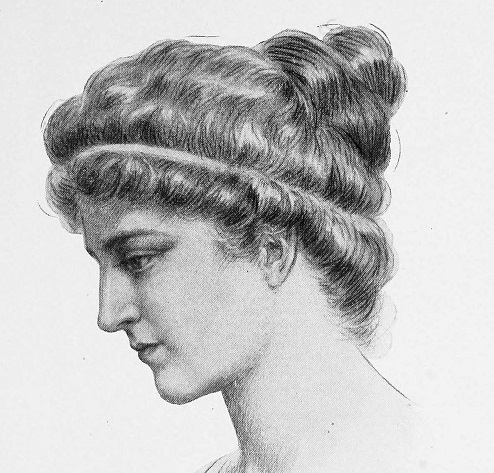She was a famous mathematician and a philosopher. She was the first woman to give importance to mathematics. She was a genius, and for many young women, she became an inspiration and encouraged them to pursue their dreams. In Alexandria's history, she was the last famous mathematician.

### 14. Antiphon

Antiphon discovered the value of Pi. This renowned mathematician was the first one who calculated the upper bound and lowers bound values of Pi by inscribing and circumscribing around a circle, the polygon, and processed finally to calculate the areas of the polygon. His idea of calculating a polygon area became very famous, and it changed mathematics for the world.

### 15. Diocles

Diocles was a profound geometer and mathematician. He was renowned for his discovery in the subdivision of geometry. In the books of Mathematics, the ‘Geometry curve’ is known by his name as the ‘Cissoid of Diocles.’ To find out a solution to doubling the cube, the method of Cissoid of Diocles was used.

## Conclusion

We have discussed above the list of famous mathematicians and their contributions to mathematics. However, they all are from Greek. There are famous Indian mathematicians also like Srinivasa Ramanujan, Aryabhata, Shakuntala Devi, and many more.

If you wish to know more about famous Indian mathematicians and their mathematics contributions, then comment in this section. For any question related to this article about ‘famous mathematicians and their contributions, ’ feel free to ask.